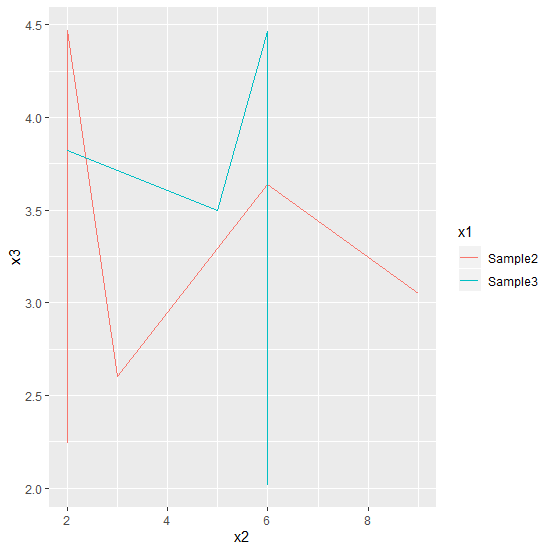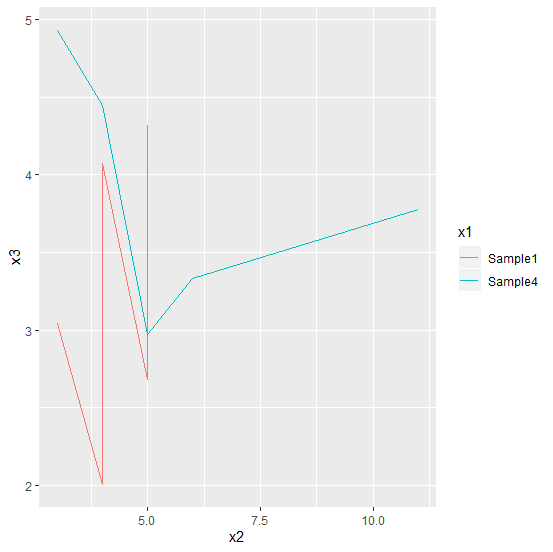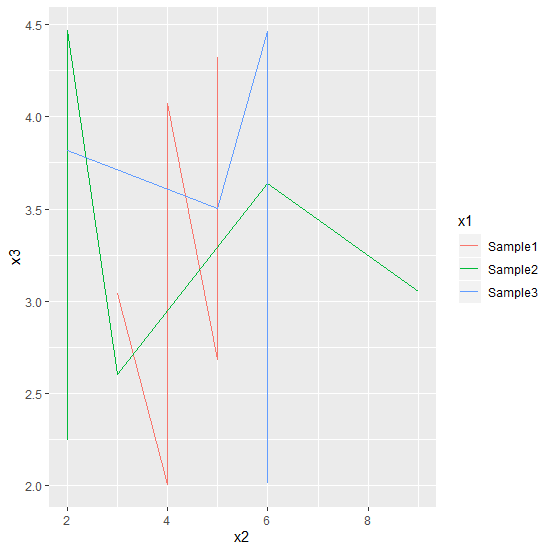# How to create a line chart for a subset of a data frame using ggplot2 in R?

Subsetting is not a difficult thing in R but if we make our code short then it is a little tedious task because we will have to introduce code between codes and that creates confusion. Therefore, we must be very careful while writing a code inside another code. To create a line with subsetting the data frame using ggplot function of ggplot2 can be done by using subset function.

## Example

Live Demo

Consider the below data frame −

set.seed(99)
x1<-rep(c("Sample1","Sample2","Sample3","Sample4"),times=5)
x2<-rpois(20,5)
x3<-runif(20,2,5)
df<-data.frame(x1,x2,x3)
df

## Output

   x1 x2 x3
1 Sample1 5 2.683710
2 Sample2 2 2.241572
3 Sample3 6 4.464855
4 Sample4 11 3.773342
5 Sample1 5 4.320167
6 Sample2 9 3.050258
7 Sample3 6 2.018184
8 Sample4 4 4.443519
9 Sample1 4 2.003538
10 Sample2 3 2.602071
11 Sample3 5 3.500318
12 Sample4 5 2.970510
13 Sample1 3 3.040351
14 Sample2 6 3.636462
15 Sample3 6 2.121470
16 Sample4 6 3.331542
17 Sample1 4 4.072941
18 Sample2 2 4.471554
19 Sample3 2 3.820360
20 Sample4 3 4.932325
library(ggplot2)
ggplot(subset(df,x1 %in% c("Sample2","Sample3")))+
+ geom_line(aes(x2,x3,group=x1,colour=x1))

## Outputggplot(subset(df,x1 %in% c("Sample1","Sample4")))+
+ geom_line(aes(x2,x3,group=x1,colour=x1))

## OutputCreating the plot for three samples (Sample1, Sample2, and Sample3) −

ggplot(subset(df,x1 %in% c("Sample1","Sample2","Sample3")))+
+ geom_line(aes(x2,x3,group=x1,colour=x1))

## Output